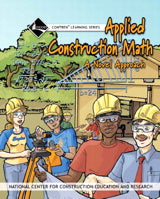# Applied Construction Math Trainee Workbook, Paperback NCCER

: In stock

: NCCER

: 9780132272988

: Book

\$40.00

#### Details

This exceptionally produced trainee guide features a highly illustrated design, technical hints and tips from industry experts, review questions and a whole lot more! NCCER introduces a new applied math book that teaches the fundamentals of math in a way that is engaging, interesting and relevant. Key content includes: Show Me the Money, It's All About Space, Where Do You Live?, Cattle Country, Breaking Ground, Payday, Divide and Conquer, Choosing Teams, Gravity Can Work for You or Against You, Shocking - Simply Shocking, First I'm Hot - Then I'm Cold, Inside and Out, The Bottom Line, and Everyone Has an Angle.

Chapter 1 Show Me the Money
Mr. Whyte is the teacher and he seizes an opportunity for a math lesson. In Chapter 1, he uses money to teach the students about decimals and factions as well as division.

Chapter 2 It's All About Space
The students meet with two potential homebuyers and teach them how to calculate the area of squares, rectangles, triangles and trapezoids.

Chapter 3 Where Do You Live?
Mr. Whyte teaches the class how to calculate the average cost of a home per square foot using powers of ten. He also explains averages.

Chapter 4 Cattle Country
Mr. Whyte finds a homebuyer! His team works with a surveyor to learn about angles, direction and bearing, the 3-4 rule for right triangles and Pythagorean Theorem, as well as more about surface area.

Chapter 5 Breaking Ground
It’s time to break ground and the class needs to learn how to estimate the volume of soil for fill and convert between units of measure.

Chapter 6 Payday
It’s payday and naturally Mr. Whyte can’t pass up an opportunity for a math lesson. This time he uses payroll taxes to teach the relationship between decimals and percentages and how to perform calculations with percentages.

Chapter 7 Divide and Conquer
The class learns how to divide the irregular shape of the foundation into common shapes so they can calculate its total volume.

Chapter 8 Choosing Teams
In this chapter, the rough carpentry team learns about linear measurements and how to read factional measurements. They also learn how to calculate beard feet and perimeters and how to estimate materials for construction.

Chapter 9 Gravity Can Work for You or Against You
Plumbers use a lot of math, so the students learn about angles, volume, pressure, grades and slopes. They also get an introduction to trigonometry.

Chapter 10 Shocking, Simply Shocking
Things are really rolling now. The electrical team learns how to calculate the circumference of a circle, more uses for the Pythagorean Theorem and more about trigonometry.

Chapter 11 First I’m Hot, Then I’m Cold
The teams reunite to install the heating and air conditioning system and to learn how to convert between the Celsius and Fahrenheit scales.

Chapter 12 Inside and Out
The kids learn how to apply math skills to finish the interior and exterior of the house. The math in this chapter includes linear measure and surface area of flat and three-dimensional objects, converting between square feet and yards, pitch and span of roofs and calculating the materials needed for a job.

Chapter 13 The Bottom Line
The job is almost done and the class learns a bit about running a financially sound business by calculating costs and determining profit.

Chapter 14 Everyone Has an Angle
This chapter wraps up trigonometry functions, including sine, casine, tangent, cotangent, secant and cosecant.

• Isbn: 9780132272988
• Publication Date: Dec 30, 2006
• Pages: 354